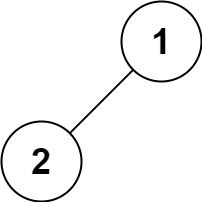## C# || How To Traverse A Binary Tree Preorder Using C#The following is a module with functions which demonstrates how to traverse a binary tree pre order using C#.

1. Binary Tree Traversal – Problem Statement

Given the root of a binary tree, return the preorder traversal of its nodes’ values.

Example 1:``` Input: root = [1,null,2,3] Output: [1,2,3] ```

Example 2:

``` Input: root = [] Output: [] ```

Example 3:

``` Input: root =  Output:  ```

Example 4:``` Input: root = [1,2] Output: [1,2] ```

Example 5:``` Input: root = [1,null,2] Output: [1,2] ```

2. Binary Tree Traversal – Solution

The following is a solution which demonstrates how to traverse a binary tree pre order.

``` 2. Binary Tree Traversal - Solution C# // ============================================================================ // Author: Kenneth Perkins // Date: Oct 13, 2021 // Taken From: http://programmingnotes.org/ // File: Solution.cs // Description: Demonstrates how to traverse a binary tree pre order // ============================================================================ /** * Definition for a binary tree node. * public class TreeNode { * public int val; * public TreeNode left; * public TreeNode right; * public TreeNode(int val=0, TreeNode left=null, TreeNode right=null) { * this.val = val; * this.left = left; * this.right = right; * } * } */ public class Solution { private List<int> result = new List<int>(); public IList<int> PreorderTraversal(TreeNode root) { Traverse(root); return result; } public void Traverse(TreeNode node) { if (node == null) { return; } result.Add(node.val); Traverse(node.left); Traverse(node.right); } }// http://programmingnotes.org/ 12345678910111213141516171819202122232425262728293031323334353637383940 // ============================================================================//    Author: Kenneth Perkins//    Date:   Oct 13, 2021//    Taken From: http://programmingnotes.org///    File:  Solution.cs//    Description: Demonstrates how to traverse a binary tree pre order// ============================================================================/** * Definition for a binary tree node. * public class TreeNode { *     public int val; *     public TreeNode left; *     public TreeNode right; *     public TreeNode(int val=0, TreeNode left=null, TreeNode right=null) { *         this.val = val; *         this.left = left; *         this.right = right; *     } * } */public class Solution {    private List<int> result = new List<int>();        public IList<int> PreorderTraversal(TreeNode root) {        Traverse(root);        return result;            }        public void Traverse(TreeNode node) {        if (node == null) {            return;        }                result.Add(node.val);                Traverse(node.left);                Traverse(node.right);    }}// http://programmingnotes.org/ ```

QUICK NOTES:
The highlighted lines are sections of interest to look out for.

The code is heavily commented, so no further insight is necessary. If you have any questions, feel free to leave a comment below.

Once compiled, you should get this as your output for the example cases:

``` [1,2,3] []  [1,2] [1,2] ```

1.FlatMajor says: